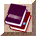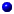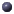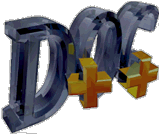##class <SatGeometry> SatGeometry : public TclObject

Library of routines involving satellite geometry

## Inheritance:

### Public Methodsstatic int are_satellites_mutually_visible(coordinate, coordinate)static double check_elevation(coordinate, coordinate, double)static double distance(coordinate, coordinate)static double get_altitude(coordinate)static double get_latitude(coordinate)static double get_longitude(coordinate)static double get_radius(coordinate a)static double propdelay(coordinate, coordinate)SatGeometry()static void spherical_to_cartesian(double, double, double, double &, double &, double &)

### Protected Methodsint command()

## Documentation

Library of routines involving satellite geometrySatGeometry()static double distance(coordinate, coordinate)static void spherical_to_cartesian(double, double, double, double &, double &, double &)static double propdelay(coordinate, coordinate)static double get_latitude(coordinate)static double get_longitude(coordinate)static double get_radius(coordinate a)static double get_altitude(coordinate)static double check_elevation(coordinate, coordinate, double)static int are_satellites_mutually_visible(coordinate, coordinate)int command()